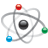## ADC Raw Values read from NTC and Current Value measured via MM9Z1J638 and

cancel
Showing results for
Did you mean:

## ADC Raw Values read from NTC and Current Value measured via MM9Z1J638 and

1,114 ViewsContributor III

Hello All,

I am studying on MM9Z1J638 and trying to understand its basics for a while. I have one question regarding to ADC values I obtained from current and temperature channels. I see 24 bit current measurement result and 16 bit raw value related to temperature. However, I do not understand what these values mean. I know that the value I read from temperature channel is dependent on the NTC I used, but is this value a voltage value, or is it something other? How I can convert, for instance the value I see from current channel to something I can understand in terms of Amps?

The demo code makes some conversions however I did not quite get how it gets temperature result form Raw ADC value. It also converts current to miliamps but I am not sure if the result I get is miliamps because what I watched on CodeWarrior is a number in Billion levels or so on. I am not sure if 12billion miliamp is something logical or not.

Labels (1)
• ### General

Tags (2)
4 Replies
508 ViewsNXP Employee

I suppose that the current reading value represents the voltage which should be converted by following equation:

And then, that voltage divided by shunt resistor value (100uOhm) to get actual current value in Amps.

The sigma-delta ADC resolution is 16-bit. Not sure if the reference voltage is 5V

I suppose we should consider the PGA gain too in this equation.

The big number as current reading you get is maybe because you are measuring negative current.

Regards,

iggi

508 ViewsContributor III

Hello Iggi,

Thank you for your response. I still have questions regarding to tthe same problem, if you let me I would like to ask one more thing. The following piece of code is taken from example code.

u32CsenseValue = (u32) B_ACQ_CURR0; // read 24bit result (two's complement 24 bit integer)
u32CsenseValue |= (u32)B_ACQ_CURR1<<16;
This shows that ADC value is kept as 32 bits rather than 24 bits which is expressed in datasheet of mm9z1j638. I think that the value in the order of 10billions is due t.o this reason. If you have more comment on this situation (its being stored as 32bit value), I am looking forward to hear.
Thanks,
508 ViewsNXP Employee

The result is 24bit and needs to be sign-extended to 32bit.

e.g.:

s32AcqCurr = (s32)B_ACQ_CURR1<<16 | (s32)B_ACQ_CURR0;

s32Current = S24SignExtend(AcqCurr);                                 // sign extend and sum up

The following macros are used:

#define S24EXTENT                 (0xFF000000UL)                        //!< macro to extend negative s24 to s32

//#define S24SignExtend(s24)     ((s24)&BIT(23))? (s24)+S24EXTENT : (s24)// works with OR and ADD

#define S24SignExtend(s24)    ((s24)&BIT(23))? (s24)|S24EXTENT : (s24)  //!< macro to sign extend s24 bit values to s32

The resolution of the B_ACQ_CURR is 0.1uV (if shunt selection is default = 100uOhm).

In this case if you use a 100uOhm shunt the resolution is one 1mA.

Regards,

iggi

508 ViewsContributor III

Hi again Iggi,

Do I need to include 32bit result to convert ADC to ampere or the last 24 bit enough in this formula Voltage = ADC_reading * (REFERENCE_VOLTAGE) / ADC_resolution